# Preface

This section is devoted to the Euler method and some of its modifications. In 1768, Leonhard Euler (pronounced "oiler" not "youler") published (St. Petersburg, Russia) an article where he introduced the tangent line method, now bearing his name. This method not only gave birth to numerical discrete methods such as Runge--Kutta, but also promoted theoretical research, including Cauchy--Lipschitz method.

# Euler's Methods

We start with the first numerical method for solving initial value problems that bears Euler's name (correct pronunciation: oiler not uler). Leonhard Euler was born 1707, in Basel, Switzerland and passed away 1783, in Saint Petersburg, Russia. In 1738, he became almost blind in his right eye. Euler was one of the most eminent mathematicians of the 18th century, and is held to be one of the greatest in history. He is also widely considered to be the most prolific mathematician of all time. He spent most of his adult life in Saint Petersburg, Russia, except about 20 years in Berlin, then the capital of Prussia.

In 1768, Leonhard Euler (St. Petersburg, Russia) introduced a numerical method that is now called the Euler method or the tangent line method for solving numerically the initial value problem:

$y' = f(x,y), \qquad y(x_0 ) = y_0 ,$
where f(x,y) is the given slope (rate) function, and $$(x_0 , y_0 )$$ is a prescribed point on the plane. Euler's method or rule is a very basic algorithm that could be used to generate a numerical solution to the initial value problem for first order differential equation. The solution that it produces will be returned to the user in the form of a list of points. The user can then do whatever one likes with this output, such as create a graph, or utilize the point estimates for other purposes. Euler's rule serves to illustrate the concepts involved in the advanced methods. It has limited usage because of the larger error that is accumulated as the process proceeds, so it requires a smaller step size if greater accuracy is desired. However, it is important to study because comprehension of Euler's method makes error analysis easier to understand.

To start, we need mesh or grid points, that is, the set of discrete points for an independent variable at which we find approximate solutions. In other words, we will find approximate values of the unknown solution at these mesh points. For simplicity, we use uniform grid with fixed step length h; however, in practical applications, it is almost always not a constant. For convenience, we subdivide the interval of interest [𝑎,b] (where usually 𝑎 = x0 is the starting point) into m equal subintervals and select the mesh/grid points

$x_n = a + n\,h \qquad\mbox{for} \quad n=0,1,2,\ldots , m; \quad\mbox{where} \quad h = \frac{b-a}{m} .$
The considered initial value problem is assumed to have a unique solution y = ϕ(x) on the interval of interest [𝑎,b], and its approximations at the grid points will be denoted by yn, so we wish that $$y_n \approx \phi (x_n ) , \quad n=1,2, \ldots .$$

There are three main approaches (we do not discuss others in this section) to derive the Euler rule: either use finite difference approximation to the derivative or transfer the initial value problem to the Volterra integral equation and then truncate it, or apply Taylor series.

1. We start with the finite difference method. It is known that numerical differentiation is an ill-posed problem that does not depend continuously on the data. If we approximate the derivative in the left-hand side of the differential equation y' = f(x,y) by the finite difference

$y' (x_n ) \approx \frac{y_{n+1} - y_n}{h}$
on the small subinterval $$[x_{n+1} , x_n ] ,$$ we arrive at the Euler's rule when the slope function is evaluated at x = xn.
\begin{equation*} y_{n+1} = y_n + (x_{n+1} - x_n ) f( x_n , y_n ) \qquad \mbox{or} \qquad y_{n+1} = y_n + h f_n , \end{equation*}
where the following notations are used: $$h=x_{n+1} - x_n$$ is the step length (which is assumed to be constant for simplicity), $$f_n = f( x_n , y_n )$$ is the value of slope function at mesh point, and yn denotes the approximate value of the actual solution y = ϕ(x) at the point $$x_n = x_0 + n\,h$$ ($$n=1,2,\ldots$$ ).
2. Integrating the identity y'(x) = f(x,y(x)) between xn and xn+1, we obtain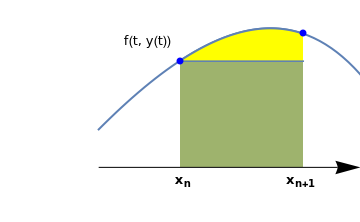curve = Plot[x - Exp[x - 0.05] + 1.5, {x, -1.0, 0.7}, PlotStyle -> Thick, Axes -> False, Epilog -> {Blue, PointSize@Large, Point[{{-0.5, 0.42}, {0.25, 0.53}}]}, PlotRange -> {{-1.6, 0.6}, {-0.22, 0.66}}]; line = ListLinePlot[{{-0.5, 0.42}, {0.25, 0.42}}, Filling -> Bottom, FillingStyle -> Opacity[0.6]]; curve2 = Plot[x - Exp[x - 0.05] + 1.5, {x, -0.5, 0.25}, PlotStyle -> Thick, Axes -> False, PlotRange -> {{-1.6, 0.6}, {0.0, 0.66}}, Filling -> Bottom, FillingStyle -> Yellow]; ar1 = Graphics[{Black, Arrowheads[0.07], Arrow[{{-1.0, 0.0}, {0.6, 0.0}}]}]; t1 = Graphics[ Text[Style["f(t, y(t))", FontSize -> 12], {-0.7, 0.5}]]; xn = Graphics[ Text[Style[Subscript["x", "n"], FontSize -> 12, FontColor -> Black, Bold], {-0.48, -0.05}]]; xn1 = Graphics[ Text[Style[Subscript["x", "n+1"], FontSize -> 12, FontColor -> Black, Bold], {0.24, -0.05}]]; Show[curve, curve2, line, ar1, t1, xn, xn1] $y(x_{n+1}) - y(x_n ) = \int_{x_n}^{x_{n+1}} f(t, y(t))\,{\text d} t .$
Such integration transfers the initial value problem for unbounded derivative operator into the integral equation, avoiding a discontinuous operator. Approximating the integral by the left rectangular (very crude) rule for numerical integration (length of interval times value of integrand at left end point) and identifying $$y(x_n )$$ with yn, we again obtain the Euler formula.

3. We may assume the possibility of expanding the solution in a Taylor series around the point xn:
$y(x_{n+1}) = y(x_n +h) = y(x_{n}) + h\, f(x_n, y(x_n )) + \frac{1}{2}\, h^2 \,y'' (x_n ) + \cdots .$
The Euler formula is the result of truncating this series after the linear term in h:    yn+1 = yn + h f(xn, yn).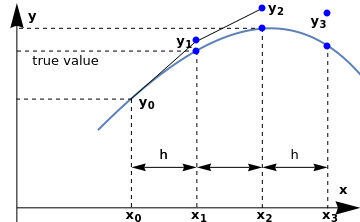curve = Plot[x - Exp[x - 0.05] + 1.5, {x, -1.0, 0.7}, PlotStyle -> Thick, Axes -> False, Epilog -> {Blue, PointSize@Large, Point[{{-0.4, 0.46}, {-0.4, 0.5}, {0, 0.63}, {0, 0.55}, {0.4, 0.48}, {0.4, 0.61}}]}, PlotRange -> {{-1.6, 0.6}, {-0.22, 0.66}}] line1 = Graphics[Line[{{-0.8, 0.27}, {-0.4, 0.5}}]]; line2 = Graphics[{Dashed, Line[{{-0.8, 0.27}, {-0.8, -0.16}}]}]; line2a = Graphics[{Dashed, Line[{{-0.8, 0.27}, {-1.5, 0.27}}]}]; line3 = Graphics[{Dashed, Line[{{-0.4, 0.46}, {-0.4, -0.16}}]}]; line3a = Graphics[{Dashed, Line[{{-0.4, 0.46}, {-1.5, 0.46}}]}]; line4 = Graphics[{Dashed, Line[{{0.0, 0.55}, {0.0, -0.16}}]}]; line4a = Graphics[{Dashed, Line[{{0.0, 0.55}, {-1.5, 0.55}}]}]; line5 = Graphics[{Dashed, Line[{{0.4, 0.48}, {0.4, -0.16}}]}]; ar1 = Graphics[{Black, Arrowheads[0.07], Arrow[{{-1.5, -0.22}, {-1.5, 0.65}}]}]; ar2 = Graphics[{Black, Arrowheads[0.07], Arrow[{{-1.5, -0.16}, {0.6, -0.16}}]}]; aa1 = Graphics[{Arrowheads[{-0.04, 0.04}], Arrow[{{-0.8, 0}, {-0.4, 0}}]}]; aa2 = Graphics[{Arrowheads[{-0.04, 0.04}], Arrow[{{-0.4, 0}, {-0.0, 0}}]}]; aa3 = Graphics[{Arrowheads[{-0.04, 0.04}], Arrow[{{0.0, 0}, {0.4, 0}}]}]; t1 = Graphics[Text[Style["h", FontSize -> 12], {-0.6, 0.05}]]; t2 = Graphics[Text[Style["h", FontSize -> 12], {-0.6, 0.05}]]; t3 = Graphics[Text[Style["h", FontSize -> 12], {0.2, 0.05}]]; x0 = Graphics[ Text[Style[Subscript["x", "0"], FontSize -> 12, FontColor -> Black, Bold], {-0.78, -0.19}]]; x1 = Graphics[ Text[Style[Subscript["x", "1"], FontSize -> 12, FontColor -> Black, Bold], {-0.38, -0.19}]]; x2 = Graphics[ Text[Style[Subscript["x", "2"], FontSize -> 12, FontColor -> Black, Bold], {0.02, -0.19}]]; x3 = Graphics[ Text[Style[Subscript["x", "3"], FontSize -> 12, FontColor -> Black, Bold], {0.42, -0.19}]]; x = Graphics[ Text[Style["x", FontSize -> 12, FontColor -> Black, Bold], {0.5, -0.09}]]; y = Graphics[ Text[Style["y", FontSize -> 12, FontColor -> Black, Bold], {-1.4, 0.6}]]; line6 = Graphics[Line[{{-0.4, 0.5}, {0.0, 0.63}}]]; y0 = Graphics[ Text[Style[Subscript["y", "0"], FontSize -> 12, FontColor -> Black, Bold], {-0.7, 0.26}]]; y1 = Graphics[ Text[Style[Subscript["y", "1"], FontSize -> 12, FontColor -> Black, Bold], {-0.47, 0.5}]]; y2 = Graphics[ Text[Style[Subscript["y", "2"], FontSize -> 12, FontColor -> Black, Bold], {0.09, 0.63}]]; y3 = Graphics[ Text[Style[Subscript["y", "3"], FontSize -> 12, FontColor -> Black, Bold], {0.35, 0.58}]]; tr = Graphics[ Text[Style["true value", FontSize -> 12, FontColor -> Black, Bold], {-1.2, 0.42}]];; Show[curve, line1, line2, line3, line2a, line3a, line4, line4a , \ line5, ar1, ar2, aa1, aa2, aa3, t1, t2, t3, x0, x1, x2, x3, x, y, \ line6, y0, y1, y2, y3,tr]
Each of these interpretations points the way to a class of generalizations of Euler's method that are discussed later. It is interesting to note that the generalization indicated by (i) which seems to be the most straightforward, has proved to be the least fruitful of the three. Therefore, Euler's rule determine next pair (xn+1, yn+1) according to the formula:

$y_{n+1} = y_n + h\, f(x_{n}, y_{n}) , \qquad y_0 = y(0), \qquad n=0,1,2,\ldots .$

Therefore, every next Euler point yn+1 is determined according to the tangent line starting from the previous grid point (xn,yn) with slope f(xn,yn); recall that the equation of the tangent line is y = yn + f(xn,yn) (x - xn). If we connect all grid points generated by the Euler algorithm

$\left( x_0 , y_0 \right) , \quad \left( x_1 , y_1 \right) , \quad \left( x_2 , y_2 \right) , \quad \cdots \quad \left( x_m , y_m \right) ,$ with straight lines, we obtain a polygonal line, called the Euler polygon, which is built up of short segments. When step size h = xn+1 - xn tends to zero, the Euler polygon approaches the solution curve. This is an essential idea of Cauchy--Lipschitz method for proving the existence theorem.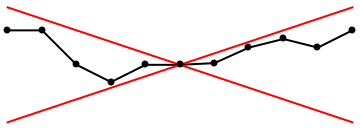euler = Plot[{x/3, -x/3}, {x, -1, 1}, Axes -> False, AspectRatio -> 1/3, PlotStyle -> {{Thick, Red}, {Thick, Red}}]; line = Graphics[{Thick, Line[{{-1, 0.2}, {-0.8, 0.2}, {-0.6, 0}, {-0.4, -0.1}, {-0.2, -0.0 .5}, {0, 0}, {0.2, 0.01}, {0.4, 0.1}, {0.6, 0.15}, {0.8, 0.1}, {1.0, 0.2}}]; point = Graphics[{PointSize[Large], Point[{{-1, 0.2}, {-0.8, 0.2}, {-0.6, 0}, {-0.4, -0.1}, {-0.2, -0.0 .5}, {0, 0}, {0.2, 0.01}, {0.4, 0.1}, {0.6, 0.15}, {0.8, 0.1}, {1.0, 0.2}}]}]; Show[line, euler, point]

Suppose that $$|f| \le M,$$ where M is a positive constant. In this case the segments in the Euler polygon have slopes between -M and M and the polygon lies between two lines of slopes ±M through the point (x0, y0). It is natural to assume that the solution has the same property. This surmise can be proved without using the Euler polygon and without assuming that the solution is unique (Cauchy--Lipschitz theorem).

This algorithm can be accomplished either directly

Clear[y]
y = 1/2; h = 0.1; f[x_, y_] = y^2 - x^2;
Do[y[n + 1] = y[n] + h f[h n, y[n]], {n, 0, 10}]
y (* to obtain the fifth numerical value *)
or using subroutine:
euler[f_,{x0_,xn_},{y0_},steps_]:=
Block[{ xold=x0,
yold=y0,
sollist={{x0,y0}},h},
h=N[(xn-x0)/steps];

Do[ xnew=xold+h;
ynew=yold+h*(f/.{x->xold,y->yold});
sollist=Append[sollist,{xnew,ynew}];
xold=xnew;
yold=ynew,
{steps}
];

Return[sollist] (* to see all grid points *)
]
Mathematica has a special subroutine to integrate using Euler's rule:
Clear[x];
x = x /. First[
NDSolve[{x'[t] == x[t]*x[t] - t^2, x == 1/2}, x, {t, 0, 1}, StartingStepSize -> 0.11,
Method -> {"FixedStep", Method -> "ExplicitEuler"}]];
grid = Table[{t, x[t]}, {t, 0, 1, 0.1}]
ListLinePlot[grid]

Example: We illustrate how the Euler method works on an example of a linear equation when all calculations become transparent. Note that Mathematica attempts to solve the ODE using its function. A solution may or may not be possible using this function. In the case when a solution may not be known or determined, Euler's method provides an alternative to approximate the solution numerically. Consider the initial value problem:

$y' = x^2 y - 1.5 \,y , \qquad y(0) =1.$
Since the given differential equation is linear, we can find its solution explicitly:
DSolve[{y'[x] == x*x*y [x] - 1.5*y[x], y == 1}, y, x]
$y = e^{1.5\,x - x^3 /3} .$
So we define its true solution in Mathematica:
true[x_] = E^(-1.5 x + 0.3333333333333333 x^3)
We substitute the slope function $$f(x,y) = x^2 y - 1.5 \,y$$ into Euler's rule to obtain
$y_{n+1} = y_n + h\,f\left( x_n , y_n \right) = y_n + h \left( x_n^2 y_n - 1.5\, y_n \right) ,$
So application of Euler's rule for the above initial value problem, we will find an approximate solution at a discrete set of nodes 0 = x0 < x1 < ··· <xN. The amount the independent variable changes each time is xn - xn-1 and is called the step size. Using the step length uniform, we get
$x_{n+1} = x_n + h = \left( n+1 \right) h$
because we start with zero: 0 = x0. The solution y(x) to the given initial value problem at the mesh point xn is estimated by yn. Factoring the slope function f(xn,yn), we get the linear difference equation of first order:
$y_{n+1} = y_n \left( 1 + h\,x_n^2 - 1.5\,h \right) = y_n \left( 1 + h^3\,n^2 - 1.5\,h \right) , \qquad n=0,1,2,\ldots ; \quad y_0 = 1.$
We discussed these equations in section, and it has the explicit solution expressed via product of all intermediate terms:
$y_{n+1} = \prod_{k=0}^n \left( 1 + h^3\,k^2 - 1.5\,h \right) , \qquad n=0,1,2,\ldots .$
Mathematica is able to solve the above difference equation, but it is represented through a special function.
sol = RSolveValue[{a[n + 1] == a[n]*(1 + n^2 *h^3 - 3/2*h), a == 1}, a[n], n]

Let us check a few first terms:

\begin{align*} y_1 &= 1 - 1.5\,h , \\ y_2 &= \left( 1 - 1.5\,h \right) \left( 1 + h^3 - 1.5\,h \right) , \\ y_3 &= \left( 1 - 1.5\,h \right) \left( 1 + h^3 - 1.5\,h \right) \left( 1 + 4\,h^3 - 1.5\,h \right) , \\ y_4 &= \left( 1 - 1.5\,h \right) \left( 1 + h^3 - 1.5\,h \right) \left( 1 + 4\,h^3 - 1.5\,h \right) \left( 1 + 9\,h^3 - 1.5\,h \right), \\ \vdots & \qquad \vdots . \end{align*}
We plot these discrete values and compare with the true solution.
h = 0.1;
DiscretePlot[sol, {n, 0, 27}, PlotStyle -> {PointSize[0.02]}]
soldif = NDSolve[{y'[x] == x^2 *y[x] - 1.5*y[x], y == 1}, y, {x, 0, 2.8}]
Plot[Evaluate[y[x]] /. soldif, {x, 0, 2.7}, PlotStyle -> {Black, Thick}, PlotRange -> {0, 3}]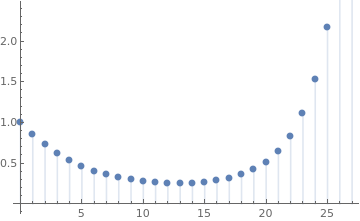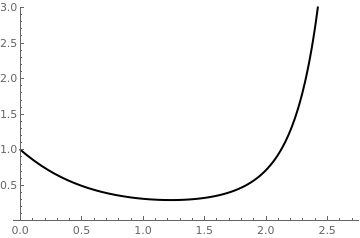Euler's discrete approximation True solution
Now we are ready to use Mathematica. First, we do preprocessing by plugging in the numerical values:
f[x_, y_] := y*x^2 - 1.5*y; (* slope function *)
x0 := 0 (* starting point in x *)
y0 := 0 (* starting value for y *)
xf=2.0; (* Value of x at which y is desired *)
h = (xf - x0)/5.0 (* step size in x *)
We attempt to find an approximation for x=2 with a step size of 0.4. Then we find the explicit expression for the formulated above IVP using standard Mathematica command and plot it:
soln = DSolve[{f[x, y[x]] == y'[x], y[x0] == y0}, y, x]
plot = Plot[Evaluate[y[x] /. soln], {x, x0, xf},
PlotStyle -> {{Thickness[0.01], RGBColor[1, 0, 0]}}]
Now we implement Euler's algorithm:
X = x0; Y = y0;
X = X + h
Y = Y + f[X, Y]*h
plot1 = ListPlot[{{X, Y}, {X, Y}}, Joined -> True,
PlotStyle -> {Thickness[0.005], RGBColor[0, 0, 1]}, DisplayFunction -> Identity]
The procedure is repeated using the next x value (x = x + h) and the y value approximation in the previous step.
X = X + h
Y = Y + f[X, Y]*h
plot2 = ListPlot[{{X, Y}, {X, Y}, {X, Y}}, Joined -> True,
PlotStyle -> {Thickness[0.005], RGBColor[0, 0, 1]}, DisplayFunction -> Identity]
The procedure on the third step is repeated using the next x value (x = x + h) and the y value approximation in the previous step.
X = X + h
Y = Y + f[X, Y]*h
plot3 = ListPlot[{{X, Y}, {X, Y}, {X, Y}, {X, Y}}, Joined -> True,
PlotStyle -> {Thickness[0.005], RGBColor[0, 0, 1]}, DisplayFunction -> Identity]
On the fourth step,we have
X = X + h
Y = Y + f[X, Y]*h
plot4 = ListPlot[{{X, Y}, {X, Y}, {X, Y}, {X, Y}, {X, Y}}, Joined -> True,
PlotStyle -> {Thickness[0.005], RGBColor[0, 0, 1]}, DisplayFunction -> Identity]
Finally, we plot Euler's approximations along with the explicit solution:
Show[plot, plot4, PlotRange -> Automatic]

In the above codes, a special option DisplayFunction was used. You can either remove this option or replace with a standard one: DisplayFunction -> $DisplayFunction. All Mathematica graphics functions such as Show and Plot have an option DisplayFunction,which specifies how the Mathematica graphics and sound objects they produce should actually be displayed. The way this works is that the setting you give for DisplayFunction is automatically applied to each graphics object that is produced. DisplayFunction ->$DisplayFunction default setting
DisplayFunction -> Identity generate no display
DisplayFunction -> f apply f to graphics objects to produce display

Within the Mathematica kernel, graphics are always represented by graphics objects involving graphics primitives. When you actually render graphics, however, they must be converted to a lower-level form which can be processed by a Mathematica front end, such as a notebook interface, or by other external programs. The standard low-level form that Mathematica uses for graphics is PostScript. The Mathematica function Display takes any Mathematica graphics object, and converts it into a block of PostScript code. It can then send this code to a file, an external program, or in general any output stream.

The results of applying Euler's rule with h = 0.1
n xn yn Exact solution Absolute error
1 0.1 0.85 0.860995 0.0109949
2 0.2 0.72335 0.742796 0.0194464
3 0.3 0.617741 0.643393 0.0256518
4 0.4 0.530639 0.560645 0.030006
5 0.5 0.459534 0.492464 0.0329305
6 0.6 0.402092 0.436922 0.0348302
7 0.7 0.356254 0.392324 0.0360707
8 0.8 0.320272 0.357245 0.0369731
9 0.9 0.292729 0.330549 0.0378206
10 1.0 0.27253 0.311403 0.0388729
■

Example: Consider the initial value problem for the logistic equation

$\frac{{\text d}y}{{\text d}t} = y \left( 1-y \right) , \qquad y(0) = y_0 =0.5.$
This problem has a unique solution
$y(t) = \frac{C\, e^t}{C\,e^t +1} , \qquad \mbox{where} \qquad C= \frac{y_0}{1-y_0} .$
Using the following Mathematica code
y = 0.5; h = 0.1
y = y + h*y*(1 - y)
Do[y[n] = y[n - 2] + 2*h*y[n - 1]*(1 - y[n - 1]), {n, 2, 500}]
ListPlot[Table[{n*h, y[n]}, {n, 0, 500}]]
we observe instability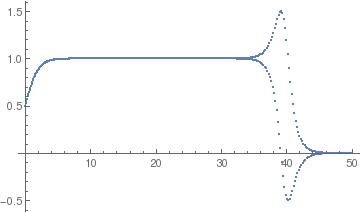Another approach is based on transferring the given IVP $$y' = f(x,y) , \quad y(x_0 ) = y_0$$ to an equivalent integral equation

$y(x_{n+1}) = y(x_n ) + \int_{x_n}^{x_{n+1}} f(s, y(s))\,{\text d} s , \qquad n=0,1,2,\ldots .$
With the application of left Riemann approximation, we come to the Euler's rule.
true[x_] = Exp[x]/(1 + Exp[x])
y = 0.5; h = 0.1; f[y_] = y^2 - y;
Do[y[n + 1] = y[n] + h f[y[n]], {n, 0, 500}]
y
The results of applying Euler's rule with h = 0.1
n xn yn Exact solution Absolute error
1 0.1 0.475 0.524979 0.0499792
2 0.2 0.450063 0.549834 0.0997715
5 0.5 0.376852 0.622459 0.245607
10 1.0 0.266597 0.731059 0.464462
20 2.0 0.114573 0.880797 0.766224
30 3.0 0.0435246 0.952574 0.90905
50 5.0 0.00552563 0.993307 0.987782
100 10.0 0.0000286528 0.999955 0.999926
200 20.0 7.61083×10-10 1. 1.
400 40.0 5.3695×10-19 1. 1.
■

Example: We consider the following initial value problem for the Riccati equation

$y' = y^2 - x^2 , \qquad y(0) = 1/2 .$
First, we find its explicit solution using mathematica standard command DSolve: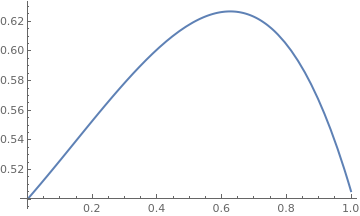explicit = DSolve[{y'[x] == y[x]^2 - x^2 , y == 1/2}, y, x] true[x_] = y[x] /. explicit Since the expression for the explicit solution involves special functions, we just evaluate its one particular value at x = 0.5 and plot the function true[0.5] {0.617769 + 2.77556*10^-17 I} Plot[true[x], {x, 0, 1}, PlotStyle -> Thick]
Now we apply Euler's rule using our subroutine euler:
euler[y^2 - x^2, {0, 1}, {0.5}, 10]
{{0, 0.5}, {0.1, 0.525}, {0.2, 0.551563}, {0.3, 0.577985}, {0.4, 0.602391}, {0.5, 0.622679}, {0.6, 0.636452}, {0.7, 0.640959}, {0.8, 0.633042}, {0.9, 0.609116}, {1., 0.565218}}
Therefore, we Euler's approximation to be 0.622679.
The results of applying Euler's rule with h = 0.1
n xn yn Exact solution Absolute error
1 0.1 0.525 0.525974 0.000973594
2 0.2 0.551563 0.552738 0.0011751
3 0.3 0.577985 0.578417 0.000432741
4 0.4 0.602391 0.600901 -0.00149051
5 0.5 0.62267 0.617769 -0.00490958
6 0.6 0.636452 0.626228 -0.0102239
7 0.7 0.640959 0.623052 -0.0179072
8 0.8 0.633042 0.604575 -0.028467
9 0.9 0.609116 0.566763 -0.0423522
10 1.0 0.565218 0.505431 -0.0597873
■

Example: To demonstrate the latter approach, we consider the initial value problem for the integro-differential equation

$\dot{y} = 2.3\, y -0.01\,y^2 -0.1\,y\, \int_0^t y(\tau )\,{\text d}\tau , \qquad y(0) =50.$
Choosing the uniform grid $$t_k = k\,h , \quad k=0,1,2,\ldots , m;$$ we integrate both sides to obtain the integral equation
$y(t ) = 50 + 2.3\,\int_0^t y(s)\,{\text d}s -0.01\,\int_0^t y^2 (s) \,{\text d}s -0.1\,\int_0^t y(s)\,{\text d}s \int_0^s y(\tau )\,{\text d}\tau$
for each mesh point t = tk. Since the double integral can be written as
$\int_0^t y(s)\,{\text d}s \int_0^s y(\tau )\,{\text d}\tau = \int_0^t \,{\text d}\tau \,\int_{\tau}^t {\text d}s \, y(s)\,y(\tau ) ,$
application of the left rectangular rule yields for the first mesh point t1 = h:
$y(t_1 ) \approx 50 + 2.3\,h\,y(0) -0.01\,h\,y^2 (0) -0.1 \, h\, \int_0^{t_1} {\text d}s \, y(s)\,y(0 ) \approx 50 +2.3\,h\,50 -0.01\,h \,50^2 - 0.1\,h^2 \,50^2 .$
So we choose the right hand-side as the approximate value y1.

For the general step in Euler's rule, we have

$y_{k+1} = y_k + 2.3\,h\,y_k -0.01\,h\,y^2_k -0.1 \, y_k \,h \int_0^{t_k} {\text d}s \, y(s)\qquad k=1,2,\ldots .$
If the trapezoidal rule is used to approximate the integral, then this expression becomes
$y_{k+1} = y_k + 2.3\,h\,y_k -0.01\,h\,y^2_k -0.1 \, y_k \,h\, T_k (h) ,\qquad k=1,2,\ldots ;$
where $$T_0 (h) = h^2 \,50^2$$ and
$T_{k} = T_{k-1} (h) + \frac{h}{2} \left( y_k + y_{k-1} \right) ,\qquad k=1,2,\ldots ;$
Finally, we ask Mathematica to perform all calculations:
h := 0.001
T := h^2 *50^2
y := 50
y := y + 2.3*h*50 - 0.01*h*50^2 - 0.1*h^2*50^2
Do[ T[k] = T[k - 1] + h*(y[k] + y[k - 1])/2;
y[k + 1] = y[k] + 2.3*h*y[k] - 0.01*h*(y[k])^2 - 0.1*y[k]*h*T[k], {k, 1, 2000}]
ListPlot[Table[{k*h, y[k]}, {k, 1, 2000}]]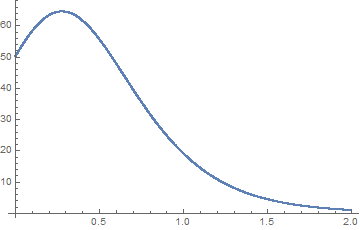■

Graphical Interpretation of the Euler rule

There are several ways to implement the Euler numerical method for solving initial value problems. We demonstrate its implementations in a series of codes. To start, define the initial point and then the slope function:

Clear[x0,y0,x,y,f]
{x0, y0} = {0, 1}
f[x_, y_] = x^2 + y^2          (* slope function f(x,y) = x^2 + y^2 was chosen for concreteness *)

Next, define the step size:

h = 0.1
Now we define the Euler method itself:
euler[{x_, y_}] = {x + h, y + h*f[x, y]}
Create the table of approximations using Euler's rule:
eilist = NestList[euler, {x0, y0}, 10]

Plot with some options:

plp = ListPlot[eilist]or
ListPlot[eilist, Joined -> True]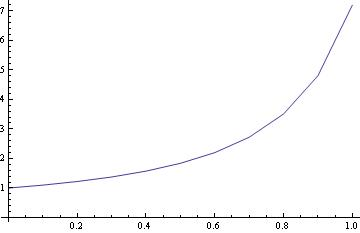or
ListPlot[eilist, Joined -> True, Mesh -> All]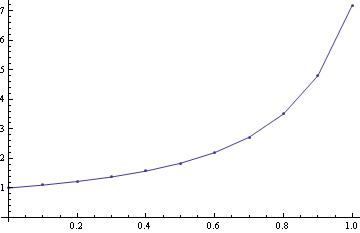or
ListPlot[eilist, Filling -> Axis]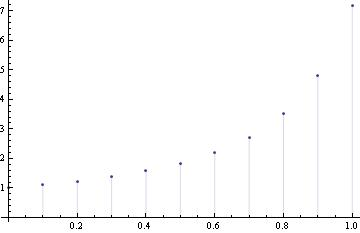Another way is to make a loop.

Clear[y]
y=1; f[x_,y_]=x^2 +y^2 ;
Do[y[n + 1] = y[n] + h f[1+.01 n, y[n]], {n, 0, 99}]
y

First of all, it is always important to clear all previous assignment to all the variables that we are going to use, so we have to type: Clear[y]

The basic structure of the loop is:
Do[some expression with n, {n, starting number, end number}]
or with option increment, denoted by k:
Do[some expression with n, {n, starting number, end number, k}]

The function of this Do loop is to repeat the expression, with n taking values from “starting number” to “ending number,” and therefore repeat the expression (1+ (ending number) - (starting number))  many times. For our example, we want to iterate 99 steps, so n will go from 0, 1, 2, ..., until 99, which is 100 steps in total. There is just one technical issue: we must have n as an integer. Therefore we let y[n] denote the actual value of y(x) when x = n*h. This way everything is an integer, and we have our nice do loop.
Finally put y , which is actually y(0.1), then shift+return, and we have our nice answer.

First, we start with the output of our program, which is, perhaps, the most important part of our program design. You'll notice that in the program description, we are told that the "solution that it produces will be returned to the user in the form of a list of points. The user can then do whatever one likes with this output, such as create a graph, or utilize the point estimates for other purposes."

Next, we must decide upon the information that must be passed to the program for it to be able to achieve this. In programming jargon, these values are often referred to as parameters. When thinking of parameters that an Euler program need to know in order to start solving the problem numerically, quite a few should come to mind:

The slope function f(x, y)
The initial x-value, x0
The initial y-value, y0
The final x-value,
The number of subdivisions to be used when chopping the solution interval into individual jumps or the step size.

To code these parameters into the program, we need to decide on actual variable names for each of them. Let's choose variable names as follows:

f, for the slope function f(x, y)
x0,      for the initial x-value, x0
y0,      for the initial y-value, y0
xn,      for the final x-value
Steps, for the number of subdivisions
h,        for step size

There are many ways to achieve this goal. However, it is natural to have the new subroutine that looks similar to built-in Mathematica's commands. So our program might look something like this:

euler[f,{x,x0,xn},{y,y0},Steps]

In order to use Euler's method to generate a numerical solution to an initial value problem of the form:

$y' = f(x, y) , \qquad y(x_0 ) = y_0 .$

We have to decide upon what interval, starting at the initial point x0, we desire to find the solution. We chop this interval into small subdivisions of length h, called step size. Then, using the initial condition $$(x_0,y_0)$$ as our starting point, we generate the rest of the solution by using the iterative formulas:

$\begin{split} x_{n+1} = x_n + h , \\ y_{n+1} = y_n + h f(x_n, y_n) \end{split}$
to find the coordinates of the points in our numerical solution. The algorithm is terminated when the right end of the desired interval has been reached.

If you would like to use a built-in Euler’s method, unluckily there is none. However, we can define it ourselves. Simply copy the following command line by line:

euler[f_, {x_, x0_, xn_}, {y_, y0_}, Steps_] :=
Block[{ xold = x0, yold = y0, sollist = {{x0, y0}}, h },
h = N[(xn - x0) /Steps];            (* or n = (xn - x0) /Steps//N; *)
Do[ xnew = xold + h;
ynew = yold + h * (f /. {x -> xold, y -> yold});
sollist = Append[sollist, {xnew, ynew}];
xold = xnew;
yold = ynew,
{Steps}
];
Return[sollist]
]

Now we have our euler function:   euler[f(x,y), {x,x0,x1},{y,y0},steps]
Then this script will solve the differential equation y’=f(x,y), subject to the initial condition y(x0)=y0, and generate all values between x0 and x1. The number of steps for the Euler’s method is specified with steps.
To solve the same problem as above, we simply need to input:

euler[1/(3*x - 2*y + 1), {x, 0, 0.4}, {y, 1}, 4]
Out= {{0, 1}, {0.1, 0.9}, {0.2, 0.7}, {0.3, 1.2}, {0.4, 1.}}

Example: Consider the initial value problem $$y' = x+y, \quad y(0) =1.$$

euler[y+x, {x, 0, 1}, {y, 1}, 10]
Out= {{0, 1}, {0.1, 1.1}, {0.2, 1.22}, {0.3, 1.362}, {0.4, 1.5282}, {0.5,
1.72102}, {0.6, 1.94312}, {0.7, 2.19743}, {0.8, 2.48718}, {0.9, 2.8159}, {1., 3.18748}}

As you can see, the output is really a big table of values, and you can just read the last one off to get y(1). If only one final value is needed, then the Return command should be replaced with
Return[sollist[[Steps + 1]]]]

We can plot the output as follows

ListPlot[euler[y + x, {x, 0, 1}, {y, 1}, 10]]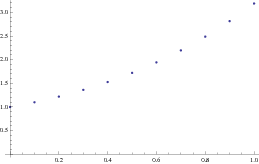Or we can plot it as

aa = euler[y + x, {x, 0, 1}, {y, 1}, 10]
Out= {{0, 1}, {0.1, 1.1}, {0.2, 1.22}, {0.3, 1.362}, {0.4, 1.5282}, {0.5,
1.72102}, {0.6, 1.94312}, {0.7, 2.19743}, {0.8, 2.48718}, {0.9,
2.8159}, {1., 3.18748}}
ListPlot[aa, AxesLabel -> {"x", "y"}, PlotStyle -> {PointSize[0.015]}]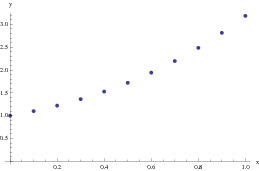The following code, which uses a slightly different programming paradigm, implements Euler's method for a system of differential equations:

euler[F_, a_, Y0_, b_, Steps_] :=
Module[{t, Y, h = (b - a)/Steps//N, i},
t = a; Y = Y0;
Do[
t[i] = a + i h;
Y[i] = Y[i - 1] + h F[t[i - 1], Y[i - 1]],
{i, 1, n}
];
Table[{t[i], Y[i]}, {i, 0, n}]
]

And the usage message is:

euler::usage = "euler[F, t0, Y0, b, n] gives the numerical solution to {Y' == F[t, Y], Y[t0] == Y0} over the interval\n
[t0, b] by the n-step Euler's method. The result is in the form of a table of {t, Y} pairs."

Note that this function uses an exact increment h rather than converting it explicitly to numeric form using Mathematica command N. Of course you can readily change that or accomplish the corresponding value by giving an approximate number for one of the endpoints.

Next we plot the points by joining them with a curve:

a = ListPlot[euler[f, 0, 1, 3, 30], Joined -> True]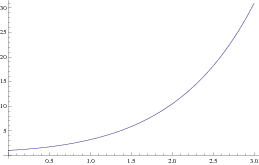Another way without writing a subroutine:

f[x_, y_] := y^2 - 3*x^2;
x0 = 0;
y0 = 1;
xend = 1.1;
steps = 5;
h = (xend - x0)/steps // N;
x = x0;
y = y0;
eulerlist = {{x, y}};
For[i = 1, i <= steps, y = f[x, y]*h + y;
x = x + h;
eulerlist = Append[eulerlist, {x, y}]; i++]
Print[eulerlist]

The results can also be visualized by connecting the points:

a = ListPlot[eulerlist, Joined -> True, Epilog -> {PointSize[0.02], Map[Point, eulerlist]}];
s = NDSolve[{u'[t] == f[x, u[t]], u == 1}, u[t], {t, 0, 1.1}];
b = Plot[Evaluate[u[x] /. s], {x, 0, 1.1}, PlotStyle -> RGBColor[1, 0, 0]];
Show[a,b]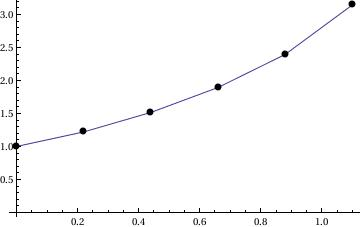Next, we illustrate application of Function command:

EulerODE[f_ /; Head[f] == Function, {t0_, y0_}, t1_, n_] :=
Module[{h = (t1 - t0)/n // N, tt, yy},
tt = t0; yy = y0;
tt[k_ /; 0 < k < n] := tt[k] = tt[k - 1] + h;
yy[k_ /; 0 < k < n] :=
yy[k] = yy[k - 1] + h f[tt[k - 1], yy[k - 1]];
Table[{tt[k], yy[k]}, {k, 0, n - 1}]
];

ty = EulerODE[Function[{t, y}, y^2/t/2 + y^2/t^1.5], {1, 1}, 2, 100];
Plot[Interpolation[ty][t], {t, 1, 2}]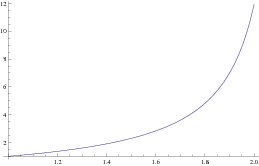Here is another steamline of the Euler method (based on Mathematica function), which we demonstrate in the following

Example: Consider the initial value problem $$y' = y^2 - 3\, x^2 , \quad y(0) =-1.$$

Clear[ x,y,h,i]
f[x_, y_] := y^2 - 3*x^2
x = 0;     y = -1;
h = 0.25; K = IntegerPart[2.5/h]
Do[ { x[i+1] = x[i]+h,
y[i+1] = y[i] +h*f[x[i],y[i]] } , {i,1,K+1} ]

Now we can plot our results, making sure we only refer to values of x and y that we have defined:

Clear[pairs]
pairs = Table[{x[i], y[i]}, {i, 1, K+1}]
plot5 = ListPlot[pairs, Joined -> True, PlotStyle -> {Thickness[0.005], RGBColor[0, 1, 0]}]
Out= {{0, -1}, {0.25, -0.75}, {0.5, -0.65625}, {0.75, -0.736084}, {1., \ -1.0225}, {1.25, -1.51113}, {1.5, -2.11213}, {1.75, -2.68436}, {2., \ -3.17979}, {2.25, -3.65202}, {2.5, -4.11458}}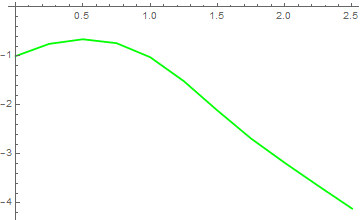Finally, we plot Euler approximations along with the actual solution:
soln = DSolve[{f[x, y[x]] == y'[x], y == -1}, y, x]
plot1 = Plot[Evaluate[y[x] /. soln], {x, 0, 2.5}, PlotStyle -> {{Thickness[0.008], RGBColor[1, 0, 0]}}];
Show[plot1, plot5]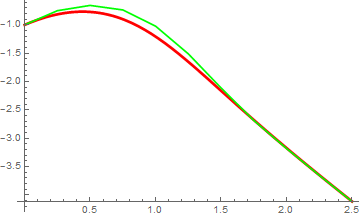Now we are going to repeat the problem, but using Mathematica lists format instead. The idea is still the same---we set a new x value, compute the corresponding y value, and save the two quantities in two lists. In particular, in a list we are simply storing values; In previously presented code, we were actually storing rules, which are more complicated to store and evaluate. We start out with just one value in each list (the initial conditions). Then we use the Append command to add our next pair of values to the list.

Clear[ x,y,h,i]
x = {0.0 };
y = {2.0 };
h = 0.1;
Do [ { x = Append[x,x[[i]]+h],
y = Append[y,y[[i]]+h*f[x[[i]],y[[i]] ] ],
} , {i,1,20} ]

Now we can plot our results:

Clear[ pairs ]
pairs = Table[ {x[[i]], y[[i]] }, {i,1,21} ]
ListPlot [ pairs, Joined -> True ]

1. Atkinson, K., Han, W., Stewart, D., Numerical Solutions of Ordinary Differential Equations, Wiley, 2009.
2. Hataue, I., Analysis of ghost numerical solutions of differential equation caused by nonlinear instability, 2012 https://doi.org/10.2514/3.12350
3. Yamaguti, M., Ushiki, S., Chaos in numerical analysis of ordinary differential equations, Physica D: Nonlinear Phenomena Volume 3, Issue 3, August 1981, Pages 618-626; https://doi.org/10.1016/0167-2789(81)90044-0